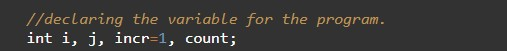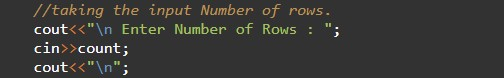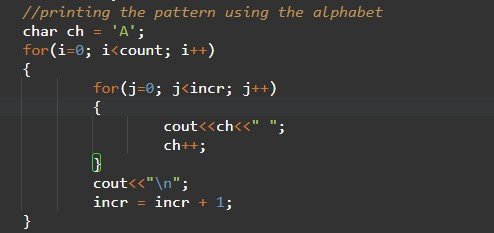# C++ Program to Print Alphabet Pattern

In this tutorial you will learn about the C++ Program to Print Alphabet Pattern and its application with practical example.

## C++ Program to Print Alphabet Pattern

In this tutorial, we will learn to create a C++ program that will Print Alphabet Pattern using C++ programming.

## Prerequisites

Before starting with this tutorial we assume that you are best aware of the following C++ programming topics:

• Operators in C++ Programming.
• Basic Input and Output function in C++ Programming.
• Basic C++ programming.
• Basic arithmetic operations.
• For loop in C++ Programming.

In C++ programming we can print many patterns with the help of codings. In today’s program, we will create the pattern using the alphabet. First, we will take the number of rows in input and then we will print the triangle of the alphabets using the for loop.

## Program:-

To Print Alphabet Pattern.

## Output:-In the above program, we have first initialized the required variable.• i = it will hold the integer value to control parent for loop.
• j = it will hold the integer value to control child for loop.
• incr  = it will hold the integer value.
• count = it will hold the integer value.

Taking the input rows from the users.Printing the patterns using the alphabet.In this tutorial we have learn about the C++ Program to Print Alphabet Pattern and its application with practical example. I hope you will like this tutorial.